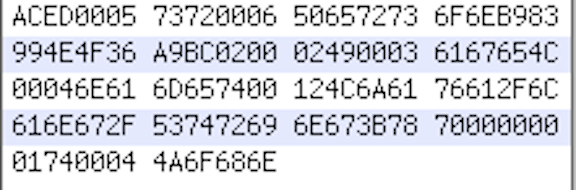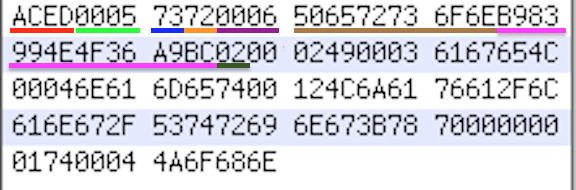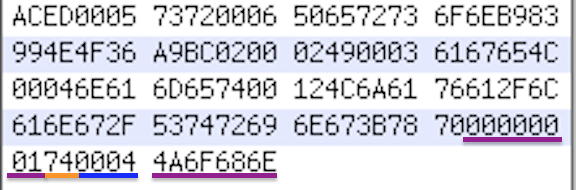effectiveJava

Effective Java - 深入解析序列化byte stream

3.再來 寫下class的instance variable的值 從最Parent class的instance開始寫

直上例子庖丁解牛ACED: MAGIC NUMBER 告訴你說這是段被序列化的byte stream

0005: STREAM_VERSION STREAM的版本

73: 新的Object

72 新的Class descriptor 接下來的是一個新的class

0006 Class name長度 (Person: 6)

506572736F6E ClassName 把16進位的值轉成ASCII

0x50 = 80 = P

0x65 = 101 = e

0x72 = 114 = r

0x73 = 115 = s

0x6F = 111 = o

0x6E = 110 = n

B983994E4F36A9BC serialVersionUID 如果你在你的Person寫死serialVersionUID 那個值就會出現在這裡

02 表示這個object支援序列化

殺進物件內部 繼續描述這個class0002: 這個物件有多少個instance (name, age 兩個)

49: 第一個variable型態 0x49 = “I” 代表integer

0003: 這個variable長度 3

616765

0x61 = 97 = a

0x67 = 103 = g

0x65 = 101 = e

4C: 這個variable型態 “L” 代表object

0004: 這個variable長度 4

6E616D65

0x6E = 110 = n

0x61 = 97 = a

0x6D = 109 = m

0x65 = 101 = e

74: 接下來是個String (TC_STRING = (byte)0x74;)

0012: 接下來這個String長度是18

4C6A6176612F6C616E672F537472696E673B:

“Ljava/lang/String;”

objectDesc:

obj_typecode fieldName className178 結束對象標誌(TC_ENDBLOCKDATA = (byte)0x78)

70 Recursive完畢 因為Person沒有父類 所以寫完一個class description就結束(TC_NULL = (byte)0x70;)00000001 第一個變數的value

74 接下來是個String (TC_STRING = (byte)0x74;)

0004: 接下來這個String長度是4

4A6F686E:

“John”